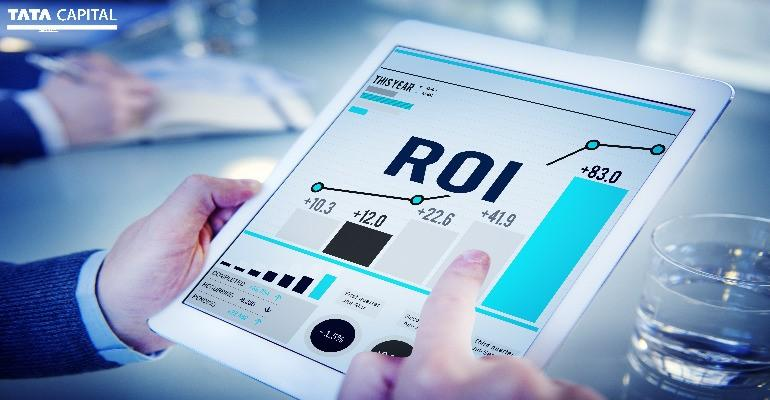Real estate has always been a sought-after investment avenue. Making the right investment decisions is easier if you can estimate profits before investing. One of the best ways to measure the efficiency of your real estate investments is Return on Investment (ROI).

## What is ROI?

ROI on real estate investments is calculated as the profits you make on the investment as a percentage of the cost. The formula for ROI is:

ROI = [(Net Income – Investment)/Investment] * 100

or

ROI = [(Net Profit)/Investment] * 100

Suppose you buy a property worth Rs. 1,00,000 and sell it at Rs. 1,50,000. Using the formula, your profit is Rs. 50,000, and your ROI is 50%.

Though the equation seems simple, there are numerous variables involved. For instance, you may spend money on maintenance and repairs or avail of a home loan. There are different ways of calculating ROI which accommodate these factors.

## Calculating ROI for cash transactions

This straightforward method of calculating ROI is called the cost method. For example, you purchased a property for Rs. 1,00,000 and spent Rs. 50,000 on its maintenance. So, your total investment is Rs. 1,50,000. If you sell the property at Rs. 2,50,000, your profit is Rs. 1,00,000, and your ROI is 66.67%.

## Calculating ROI for financed transactions

Calculating ROI on purchased homes financed with a loan involves more variables.

Suppose you bought the same property as above for Rs. 1,00,000 with a housing loan. Let’s look at the expenses.

• The down payment was 20% of the purchase price, which is Rs. 20,000
• The foreclosure cost was 4.5% of the principal amount, i.e., Rs. 4500
• You paid Rs. 50,000 for maintenance
• Your total investment is the sum of these expenses, i.e., Rs. 1,74,500

If you sell the property for Rs. 2,50,000, then:

• Your net profit will be Rs. 75,500
• Your ROI will be 43.26%

Suppose, instead of selling this property, you rent it for Rs. 20000 per month for one year.  Now you have to incur charges like electricity bill, water bill etc. which add up to Rs. 1,000 per month.  Additionally, your loan has a 4% interest rate and a tenure of 10 years, making the EMI amount Rs 1,842.

• Your monthly income is Rs. [20,000-(1,842+1,000)] i.e., Rs. 17,158
• Over 12 months, you will earn Rs. 2,05,896
• Your net annual profit is Rs. 31,396
• Your ROI over the year is 17.99%

## What is a good ROI?

The ROI on housing sector fluctuates with market conditions, but you can invest after understanding the recent trends. If you wish to buy a rental property, the ideal ROI is between 8-10%. An ROI of 12% and above is considered excellent by the industry experts.